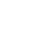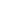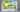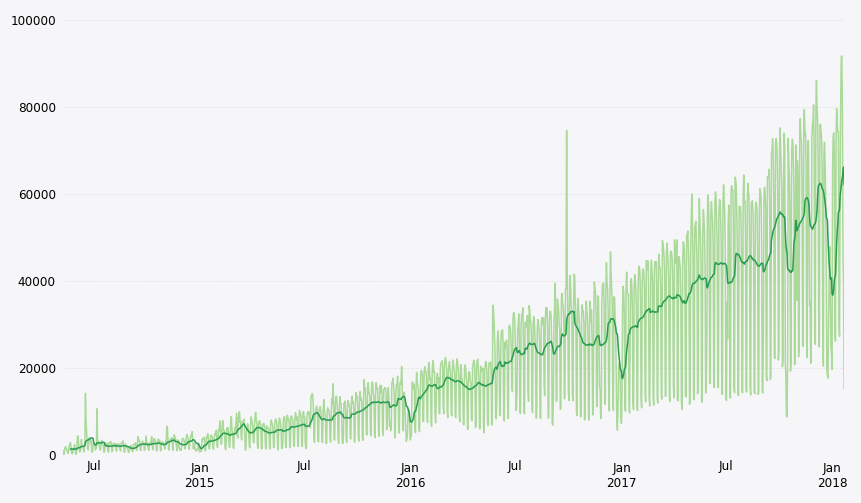Introducing Visual Explorer, a new tool for data visualization.Learn more# Window Functions in Python and SQLDavid Wallace, Data Science Evangelist

January 23, 2018 8 minute readAs an analyst or data scientist, it’s becoming increasingly advantageous to have a deep understanding of multiple analytical programming languages. There's a growing number of people using SQL and Python in a hybrid-fashion for data analysis. But, the dialogue around the usage of these two languages tends to portray them as complementary, but functionally discrete.

This dialogue fails to address the sizable middle-ground of shared functionality between SQL and Python, particularly since the advent of libraries like pandas. Our goal for this series is to shift our mindset away from thinking about them in terms of how they are different, and more in terms of how they are alike. We'll do this by relating common analytical operations in the two languages.

Exploring this middle ground can help improve our understanding of the capabilities of both languages. When we understand how the languages overlap, we can make smarter decisions about which to use and when.

With this idea in mind, let's examine a tool that most of us probably use on a day-to-day basis: Window Functions.

## From the Window, to the Window

Window functions are incredibly common operations in the world of reporting and analytics. The PostgreSQL documentation does an excellent job of introducing the concept of Window Functions:

A window function performs a calculation across a set of table rows that are somehow related to the current row. This is comparable to the type of calculation that can be done with an aggregate function. But unlike regular aggregate functions, use of a window function does not cause rows to become grouped into a single output row — the rows retain their separate identities. Behind the scenes, the window function is able to access more than just the current row of the query result.

What window functions often look like in practice is calculating a moving average from raw time series data. When displayed alongside the time series, analysts can use the moving average to emphasize a trend:### Window Functions in SQL

A SQL window function will look familiar to anyone with a moderate amount of SQL experience. At its core, A SQL window function consists of five main components:

1. The function being performed (e.g. `sum()`, `avg()`, `count()`, etc.)
2. An `over` clause immediately following the function name and arguments. This clause designates that the function being applied is a window function and should be computed across an appropriate set of rows.
3. A `partition by` list within the `over()` clause that divides the rows being processed into groups, or partitions, that share the same values in the `partition by` list. For each row, the window function is computed across the rows that fall into the same partition as the current row.
4. An `order by` list within the `over()` clause that specifies the order in which the rows should be processed.
5. A window frame clause within the `over()` clause that specifies the subset of the partition over which to operate. This can be the same size as the partition or smaller.

Taken together, this calculation will look like the following:

``````sum(<column1>) over (partition by <column2> order by <column3> rows between 13 preceding and current row) as alias
``````

This statement is the equivalent of saying “take the sum of column 1 for each distinct value in column 2, but limit this sum to the values in the current row and previous 13 rows after being ordered by column 3”.

In most SQL-based analytical data warehouses, there are specialized functions outside of the usual suspects that can be used in window functions. Examples of this include functions such as `lag()` and `lead()`, which allow you to read data from the previous or following row in the partition, respectively. Another example is `row_number()`, which returns the current row number of the query result over the window.

To provide a practical example of how SQL window functions can be applied, check out this example report where we analyze San Francisco bike share trip data. Window functions calculate measures such as a 14-day moving average, running total, week-over-week difference, and week-over-week percent increase in trips. The `Trips - SQL Window` query shows exactly how we go about doing this: we first aggregate the number of rideshare trips per day in a CTE called `input` :

``````with input as (
select
count(1) as trips,
date_trunc('day',start_date) as date
from modeanalytics.sf_bike_share_trip
group by 2
)
``````

The output of this CTE will contain the total number of trips started per day. We will apply our window function to this output. Since we are looking to calculate the moving *average *of trips, we will start by writing the function we want to perform:

``````select
date,
trips,
avg(trips) as function
from input
``````

In its current form, SQL thinks that you are trying to perform a normal `avg()` aggregation on the `trips` column. However, we want to specifically perform a window function. To tell SQL that this function should be applied as a window function, we need to follow our `avg()` function with an `over` clause:

``````select
date,
trips,
avg(trips) over () as window_function
from input
``````

At this point, we've told SQL to treat this `avg()` function as a window function. We can now begin refining our window function to suit our specific needs. Since we are looking to calculate a 14-day moving average, and each row represents a single day, we want to limit the number of rows we are processing to the current row and the previous 13:

``````select
date,
trips,
avg(trips) over (order by date rows between 13 preceding and current row) as mvg_avg
from input
``````

And that's it. We've now calculated our 14-day moving average using SQL. At this point, we have everything we need to start creating visualizations. As an example, we’ve created a chart to visualize the 14-day moving average ride share trips on top of the daily data. This visualization gives us a much better immediate understanding of the underlying trends in this data.

In the example report, we've included a few other common window operations to showcase additional windowing functionality.

But what if we wanted to use Python instead? How would the details of the implementation differ?

### Window Functions in Python

While semantically quite different, window functions in pandas share quite in a bit in common, functionality-wise, with SQL.

The way that pandas implements window functions is mainly through the operators `rolling` and `expanding`. When called on a pandas Series or Dataframe, they return a `Rolling` or `Expanding` object that enables grouping over a rolling or expanding window, respectively.

As an example, we are going to use the output of the `Trips - Python Window` query as an input to our Dataframe (`df`) in our Python notebook.

Notice that this Dataframe does not have any of the window functions being calculated via SQL. It’s simply the CTE portion of the previously used `Trips - SQL Window` query.

In our Python notebook, we are going to create a new column `mvg_avg` in our Dataframe that represents the equivalent of the 14-day moving average we previously calculated using SQL. To do this using pandas, we first select the column we want to apply our window function on (`trips`) from our Dataframe as a Series object by using `df.trips`.

We then call the `rolling` operator on the Series extracted using `df.trips` to create a new Rolling object. This Rolling object behaves similarly to a GroupBy object, except instead of enabling grouping by specific values, it enabled grouping based a sliding window defined by the user. The `rolling()` method accepts additional arguments from the user, such as:

1. `window`: the size of the moving window
2. `win_type`: the type of window to be applied.
3. `min_periods`: the threshold of non-null data points to require (default is NA)
4. `center`: whether to set the labels at the center (default is False)

In our example, we want to calculate a 14-day moving average on our daily Series using the `rolling()` method, so we will supply a value of 14 for `window`:

``````df.trips.rolling(window=14)
``````

Which can also be written in shorthand as:

``````df.trips.rolling(14)
``````

Now that we’ve created our Rolling object with a 14-day window, we are able to call any of the available methods and properties, including methods such as `mean()`, `count()`, and `median()`, amongst others. Since our goal is to calculate the 14-day moving average, we will call the `mean()` method on our Rolling object:

``````df.trips.rolling(14).mean()
``````

We can then assign our 14-day moving average to a new column in our existing Dataframe:

``````df[“mvg_avg”] = df.trips.rolling(14).mean()
``````

And that’s it! We’ve calculated and stored our 14-day moving average in our Dataframe. Compare this operation to it’s SQL equivalent:

``````avg(trips) over (order by date rows between 13 preceding and current row) as mvg_avg
``````

The pandas `.rolling()` method allows users to customize their aggregation methods even further, allowing users to specify things such as custom weighting of values in the window by using different window types. For example, we can calculate a 14-day moving average using a triangular window by supplying a `win_type` keyword argument in our `.rolling()` method:

``````df["mvg_avg_triang"] = df.trips.rolling(window=14, win_type='triang').mean()
``````

Once users overcome the nuances of how to apply window functions to Series and Dataframes in pandas, they typically come to appreciate the brevity and deep customization options it provides. The following snippets show more examples of equivalent window functions between SQL and pandas. It also showcases some syntactic sugar offered by pandas for very common window-style operations, including running totals, row differences, and percent changes between rows.

### Running Total

In SQL:

``````sum(trips) over (order by date rows unbounded preceding) as running_total_trips
``````

In Python

``````df["running_total_trips"] = df.trips.cumsum()
``````

### Week-over-week Percent Change

In SQL:

``````(trips - lag(trips,7) over (order by date))/lag(trips,7) over (order by date)::decimal(18,2) as wow_percent_change
``````

In Python:

``````df["wow_percent_change"] = df.trips.pct_change(7)
``````

### Week-over-week Difference

In SQL:

``````trips - lag(trips,7) over (order by date) as wow_difference
``````

In Python:

``````df["wow_difference"] = df.trips.diff(7)
``````

## Blur the Line

No fixed rule can determine which language should be used for which function. Everyone will have different needs. For example, some of our users have started moving operations like window functions into the Python notebook to offload work from their data warehouse. Others prefer to consolidate work in one language, so they use SQL. Only by understanding the strengths of each can you effectively wield the power of both.

That's the benefit of being able to use multiple data analysis languages; you can customize a hybrid approach to suit your needs as they evolve.

# Get our weekly data newsletter

Work-related distractions for every data enthusiast.